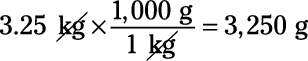##### Physics I Workbook For Dummies with Online PracticePhysics problems frequently ask you to convert between different units of measurement. For example, you may measure the number of feet your toy car goes in three minutes and thus be able to calculate the speed of the car in feet per minute, but that’s not a standard unit of measure, so you need to convert feet per minute to miles per hour, or meters per second.

For another example, suppose you have 180 seconds — how much is that in minutes? You know that there are 60 seconds in a minute, so 180 seconds equals three minutes. Here are some common conversions between units:

• 1 m = 100 cm = 1,000 mm (millimeters)

• 1 km (kilometer) = 1,000 m

• 1 kg (kilogram) = 1,000 g (grams)

• 1 N (newton) = 105 dynes

• 1 J (joule) = 107 ergs

• 1 P (pascal) = 10 Ba

• 1 A (amp) = 0.1 Bi

• 1 T (tesla) = 104 G (gauss)

• 1 C (coulomb) = 2.9979 x 109 Fr

The conversion between CGS and MKS almost always involves factors of 10 only, so converting between the two is simple. But what about converting to and from the FPI and other systems of measurement? Here are some handy conversions that you can come back to as needed:

• Length:

• 1 m = 100 cm

• 1 km = 1,000 m

• 1 in (inch) = 2.54 cm

• 1 m = 39.37 in

• 1 mile = 5,280 ft = 1.609 km

• 1 angstrom = 10–10 m

• Mass:

• 1 kg = 1,000 g

• 1 slug = 14.59 kg

• 1 u (atomic mass unit) = 1.6605 x 10–27 kg

• Force:

• 1 lb (pound) = 4.448 N

• 1 N = 105 dynes

• 1 N = 0.2248 lb

• Energy:

• 1 J = 107 ergs

• 1 J = 0.7376 ft-lb

• 1 BTU (British thermal unit) = 1,055 J

• 1 kWh (kilowatt hour) = 3.600 x 106 J

• 1 eV (electron volt) = 1.602 x 10–19 J

• Power:

• 1 hp (horsepower) = 550 ft-lb/s

• 1 W (watt) = 0.7376 ft-lb/s

Because conversions are such an important part of physics problems, and because you have to keep track of them so carefully, there’s a systematic way of handling conversions: You multiply by a conversion constant that equals 1, such that the units you don’t want cancel out.

## Sample question

1. A ball drops 5 meters. How many centimeters did it drop?

The correct answer is 500 centimeters. To perform the conversion, you do the following calculation: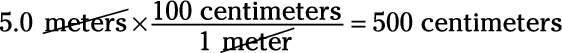Note that 100 centimeters divided by 1 meter equals 1 because there are 100 centimeters in a meter. In the calculation, the units you don’t want — meters — cancel out.

## Practice questions

1. How many centimeters are in 2.35 meters?

2. How many seconds are in 1.25 minutes?

3. How many inches are in 2.0 meters?

4. How many grams are in 3.25 kg?

Following are answers to the practice questions:

1. 235 cm

Convert 2.35 meters into centimeters: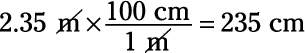2. 75 sec

Convert 1.25 minutes into seconds: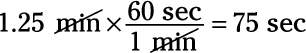3. 79 in

Convert 2.0 meters into inches: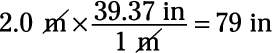4. 3,250 g

Convert 3.25 kilograms into grams: